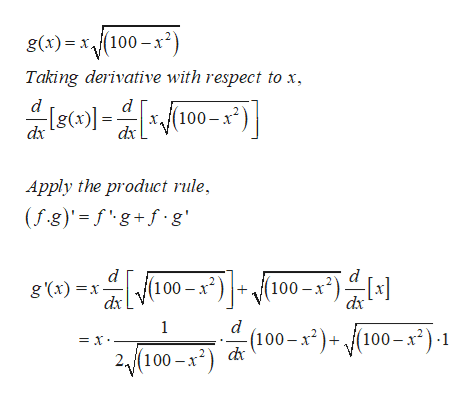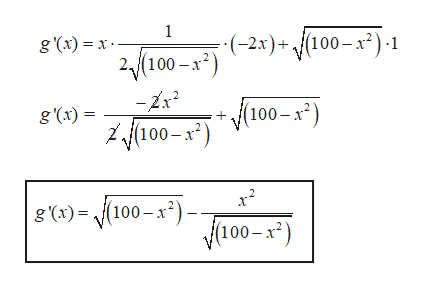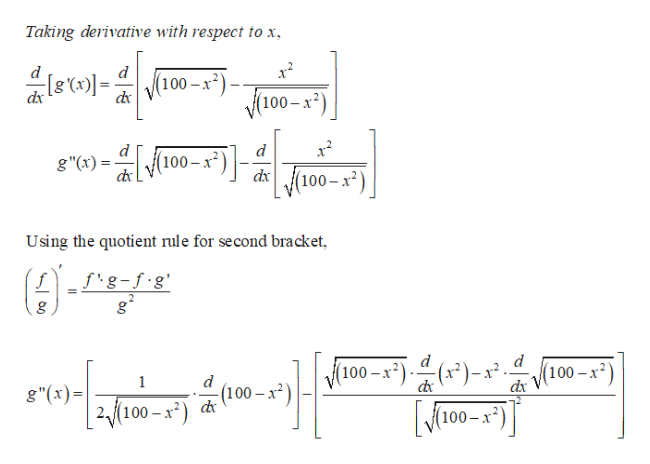# Find the derivative and second derivative of g(x)=x(100-x^2)^(1/2).

Question

Find the derivative and second derivative of g(x)=x(100-x^2)^(1/2).

check_circleExpert Solution
Step 1

Since the placement of the radical sign is not clear whether it includes x or no, the question has been solved considering the below given expression.

First derivative,help_outlineImage Transcriptioncloseg(x)x(100-x2 Taking derivative with respect to x d (100-x) dx dx Apply the product rule, (fg)' fg+f.g d 100-x2(100-x2) g x)x dx dx d 1 (100-x2)+/(100- x2)-1 = x 2(100-x2) fullscreen
Step 2

Simplify the expression,help_outlineImage Transcriptionclose1 (-2х)+ 100-х?): g () — х.— 2,(100- х*) -2x2 (100-х) g(x)= f100-х") х? |8 ()- 100-х). (100-х ) fullscreen
Step 3

Second deriva...help_outlineImage TranscriptioncloseTaking derivative with respect to x d d (100-x2) Y100-x dx g")10 (100-x d 100-x dx Using the quotient rule for second bracket f fig-f.g' (100-x:)·은(12)-x 100-x2 dx d 1 (100-x2) d g"(x) 2{100-x-") 100 fullscreen

### Want to see the full answer?

See Solution

#### Want to see this answer and more?

Solutions are written by subject experts who are available 24/7. Questions are typically answered within 1 hour*

See Solution
*Response times may vary by subject and question
Tagged in

### Derivative